# Solve Exponential Equations Questions with Solutions

How to solve exponential equations? Questions with detailed solutions for grade 12.
In what follows, exponential equations are solved analytically using the powerful method of substitution and the rules of exponential and logarithmic functions. The same equations are also solved graphically.
To solve graphically an equation of the form f(x) = g(x), we rewrite it such that the right hand side is equal to zero as follows: f(x) - g(x) = 0. We then graph the left side of the equation f(x) - g(x) and the solutions of the given equation are represented by the x intercepts of the graph.

## Solve Exponential Equations

1. Solve the equation: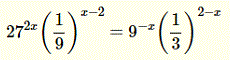Solution
Note that 27, 9 and 3 may be written as powers of 3 as follows:
27 = 33 , 9 = 32 and 3 = 31
Using the above and also the formula for negative exponentwe rewrite the given equation as follows:
(33)2x (3-2)x - 2 = (32)-x (3-1)2 - x
We now use the formula (xm)n = x m n to rewrite the above equation as follows.
36x 3-2x + 4 = 3-2x 3- 2 + x
Use the formula xm xn = xm + n to rewrite the equation as follows
36x- 2x + 4 = 3- 2x - 2 + x
Simplify the exponents to obtain
34x + 4 = 3- x - 2
We use the fact that an exponential function of the form ax is a one to one function, meaning that if 3m = 3n, then m = n to write an algebraic equation using the above equation:
4x + 4 = - x - 2
Solve the above for x to obtain the solution of the given equation.
5x = - 6 gives the solution x = - 6 / 5 = - 1.2
Below is shown the graph of the left side of the given equation when written with right side equal to zero as follows:The x intercept is an approximation to the analytical solution found above. Check that they are close in value.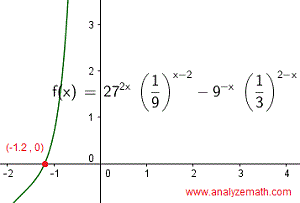.

2. Find the solutions to the equation.
Solution
Note that √(16x) = (16x)1/2 = 16(1/2)x and use it to rewrite the given equation as
16(1/2)x = (1/2)x2-1
Note that 1/2 = 2-1 and 16 = 24 and use it to rewrite the above equation as
(24)(1/2)x = (2-1)x2-1
Use the formula (xm)n = x m n to rewrite the above equation as
22x = 2-x2 + 1
We use the fact that an exponential function of the form ax is a one to one function to write.
2x = - x2 + 1
Rewrite in standard form and solve the above quadratic equation.
x2 + 2x - 1 = 0
? = 22 - 4 (1)(-1) = 8
Two solutions: x1 = - 1 - √ 2 ? -2.41 and x2 = - 1 + √ 2 ? 0.41
The graph of the left side of the given equation when written with right side equal to zero as follows: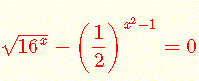is shown below. The x intercept are approximations to the solutions analytical solutions found above. Check that they have close values..

3. What are the solutions to the equation

2 x - 6 · 2 - x = 6 ?

Solution
Let u = 2x which also gives 1/u = 2- x and rewrite the equation in terms of u.
u - 6/u = 6
u cannot be zero (because 2x cannot be less than or equal to zero), we therefore multiply all terms of the above equation by u and simplify
u(u - 6/u) = 6 u
u2 - 6 = 6 u
u2 - 6u - 6 = 0
Solve for u
? = (-6)2 - 4(1)(-6) = 60
Two solutions: u1 = 3 + √15 and u2 = 3 - √15
We now solve for x using the above substitution u = 2x.
First equation: 2x = 3 + √15
ln(2x) = ln(3 + √15)
x ln(2) = ln(3 + √15)
Solution: x = ln(3 + √15) / ln 2 ? 2.78
Second equation: 2x = 3 - √15 has no solution because 3 - √15 is less than zero.
Below is shown the graph of the left side of the given equation when written with right side equal to zero as follows: 2 x - 6 · 2 - x - 6 = 0 . The x intercept is an approximation to the solution of the analytical solution found above..

4. Find the solutions to the equation

5 x+1 = 100 · 3x

Solution
Take the natural logarithm (ln) of both sides
ln (5x + 1) = ln (100 ? 3x )
Use log rules to simplify.
(x + 1) ln 5 = ln 100 + x ln 3
Expand left side and group terms with x.
x ln 5 + ln 5 = ln 100 + x ln 3
x (ln 5 - ln 3) = ln 100 - ln 5
x = (ln 100 - ln 5) / (ln 5 - ln 3) ? 5.86
Below is shown the graph of the left side of the given equation when written with right side equal to zero as follows: 5 x+1 - 100 · 3x = 0 . The x intercept is an approximation to the solution of the analytical solution found above..

5.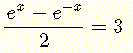.
Solution
Let u = ex which also gives 1/u = e- x and rewrite the equation in u. (u = ex cannot be less than or equal to zero)
(u - 1/u) / 2 = 3
Multiply both sides by 2 and simplify
u - 1 / u = 6
Multiply both sides by u and simplify
u2 - 1 = 6 u
u2 - 6u - 1 = 0
Solve for u
? = (-6)2 - 4(1)(-1) = 40
Two solutions: u1 = 3 + √10 and u2 = 3 - √10
We now solve for x using the above substitution u = ex.
First equation: ex = 3 + √10
ln(ex) = ln(3 + √10)
Solution: x = ln(3 + √10) ? 1.82
Second equation: ex = 3 - √10 has no solution because 3 - √10 is less than zero.
Below is shown the graph of the left side of the given equation when written with right side equal to zero as follows: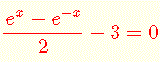. The x intercept is an approximation to the solution of the analytical solution found above..

6. What are the solutions to the equation

3 2 - 3x = 4 2x + 1

Solution
Take the natural logarithm (ln) of both sides
ln (32 - 3x) = ln (42x + 1)
Use log rules to simplify.
(2 - 3x) ln 3 = (2x + 1) ln 4
Expand both sides of the equation and group terms with x.
2 ln 3 - 3 x ln 3 = 2 x ln 4 + ln 4
x (-3 ln 3 - 2 ln 4) = ln 4 - 2 ln 3
x = (2 ln 3 - ln 4) / (3 ln 3 + 2 ln 4) ? 0.13
Below is shown the graph of the left side of the given equation when written with right side equal to zero as follows: 3 2 - 3x - 4 2x + 1 = 0. The x intercept is an approximation to the solution of the analytical solution found above..

7. Solve for x the equation:

9 x - 3 x + 1 + 2 = 0

Solution
Note that
9x = (32)x = 32 x = (3x)2
Rewrite the given equation using 9x = (3x)2 and also 3x + 1 = 3?3x
(3x)2 - 3?3x + 2 = 0
Let u = 3x and rewrite the equation in u.
u2 - 3 u + 2 = 0
Solve for u by factoring
(u - 2)(u - 1) = 0
Two solutions: u1 = 2 and u2 = 1
We now solve for x using the above substitution u = 3x.
First equation: 3x = 2
ln( 3x) = ln 2
x ln 3 = ln 2
Solution: x = ln 2 / ln 3 ? 0.63
Second equation: 3x = 1 , solution x = 0.
Below is shown the graph of the left side of the given equation. The x intercepts are approximations to the analytical solutions found above..